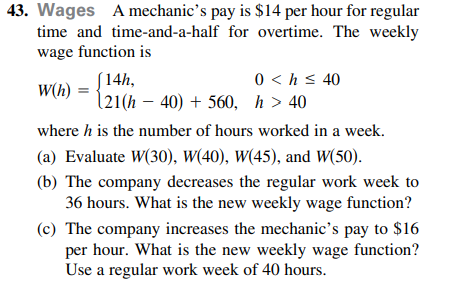### Still have math questions?

Algebra
QuestionWages A mechanic's pay is $$\ 14$$ per hour for regular time and time-and-a-half for overtime. The weekly wage function is

$$W ( h ) = \left\{ \begin{array} { l l } { 14 h , } \\ { 21 ( h - 40 ) + 560 , h > 40 } \end{array} \right.$$

where $$h$$ is the number of hours worked in a week.

(a) Evaluate $$W ( 30 ) , W ( 40 ) , W ( 45 )$$ , and $$W ( 50 ) .$$

(b) The company decreases the regular work week to $$36$$ hours. What is the new weekly wage function?

(c) The company increases the mechanic's pay to $$\ 16$$ per hour. What is the new weekly wage function? Use a regular work week of $$40$$ hours.